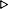ToC DocOverview CGDoc RelNotes Index PermutedIndex
Allegro CLANSI Common Lisp5 Data and Control Flow5.3 Dictionary of Data and Control Flow

 5.3.45 when, unless Macro

Syntax:
when test-form {form}*{result}*

unless test-form {form}*{result}*

Arguments and Values:
test-form - a form.

forms - an implicit progn.

results - the values of the forms in a when form if the test-form yields true or in an unless form if the test-form yields false; otherwise nil.

Description:
when and unless allow the execution of forms to be dependent on a single test-form.

In a when form, if the test-form yields true, the forms are evaluated in order from left to right and the values returned by the forms are returned from the when form. Otherwise, if the test-form yields false, the forms are not evaluated, and the when form returns nil.

In an unless form, if the test-form yields false, the forms are evaluated in order from left to right and the values returned by the forms are returned from the unless form. Otherwise, if the test-form yields false, the forms are not evaluated, and the unless form returns nil.

Examples:
``` (when t 'hello)HELLO
(unless t 'hello)NIL
(when nil 'hello)NIL
(unless nil 'hello)HELLO
(when t)NIL
(unless nil)NIL
(when t (prin1 1) (prin1 2) (prin1 3))1233
(unless t (prin1 1) (prin1 2) (prin1 3))NIL
(when nil (prin1 1) (prin1 2) (prin1 3))NIL
(unless nil (prin1 1) (prin1 2) (prin1 3))1233
(let ((x 3))
(list (when (oddp x) (incf x) (list x))
(when (oddp x) (incf x) (list x))
(unless (oddp x) (incf x) (list x))
(unless (oddp x) (incf x) (list x))
(if (oddp x) (incf x) (list x))
(if (oddp x) (incf x) (list x))
(if (not (oddp x)) (incf x) (list x))
(if (not (oddp x)) (incf x) (list x))))((4) NIL (5) NIL 6 (6) 7 (7))
```

and, cond, if, or

Notes:
``` (when test {form}+) ==(and test (progn {form}+))
(when test {form}+) ==(cond (test {form}+))
(when test {form}+) ==(if test (progn {form}+) nil)
(when test {form}+) ==(unless (not test) {form}+)
(unless test {form}+) ==(cond ((not test) {form}+))
(unless test {form}+) ==(if test nil (progn {form}+))
(unless test {form}+) ==(when (not test) {form}+)
```

Allegro CL Implementation Details:
None.© Franz Inc. 1998-2019 - File last updated 02-07-2019